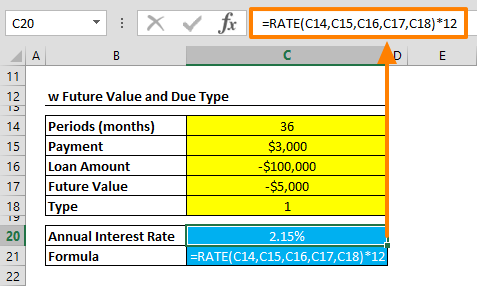The Excel RATE function is a Financial formula that calculates and returns the interest rate for a loan, based on constant payments and interest rate. In this guide, we’re going to show you how to use the Excel RATE function and also go over some tips and error handling methods.

## Supported versions

• All Excel versions

## Excel RATE Function Syntax

RATE(nper, pmt, pv, [fv], [type], [guess])

## Arguments

 nper The total number of payments for the loan. pmt The constant payment amount. pv The present value, or total value of all loan payments today. [fv] Optional. The future value, or cash balance you want after the last payment is made. Default value is 0 (zero). [type] Optional. When payments are due. 0 = end of period. (Default) 1 = beginning of period. [guess] Optional. Your guess on the rate, can be a value between 0 and 1. The default is 10%.

## Examples

### Without future value and due date type

The RATE function has 3 required arguments: nper, pmt and pv. Using these arguments, you can calculate the payment rate through the loan period. The function assumes that payments are made at the end of each period, and the loan is to be paid in full.

For example, the following formula calculates the annual rate for a loan of \$100,000 over 36-months with constant payments of \$3,000.

=RATE(36,3000,-100000)*12

Please note that, the result is multiplied by 12, because the interest rate is given as an annual rate, while periods are in months.### With future value and due date type

You can specify a target cash balance for after the last payment is made, and whether payments are to be made at the beginning of each period or the end.

The following formula is the extended version of the first example. It calculates same amount for the same period with the same interest. The difference is that there is \$5,000 left after the last payment, and the payments are made at the beginning of each period.

=RATE(36,3000,-100000,-5000,1)*12## Tips

• The RATE function does iterative calculations to calculate the rate. There may be zero or multiple solutions. The function checks whether the results converge within 0.0000001 after 20 iterations.
• If pv is entered as a positive number, the pmt should be negative, or vice versa. This is because the money is "coming out of pocket".
• If nper is given in months and you want to find the annual rate, multiply the return value of RATE with 12.
• Use the [guess] argument (try different values than 10%) if return value does not converge.
• Make sure that you enter consistent values in terms of  units when entering [guess] and nper. If there are monthly payments on a 5-year loan at 6% annual interest, use 6%/12 for [guess] and 5*12 for nper.
• Other related financial functions:

## Issues

• If the return value doesn't converge within 0.0000001 precision after 20 iterations, the function returns #NUM!